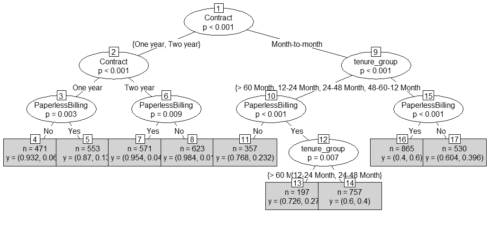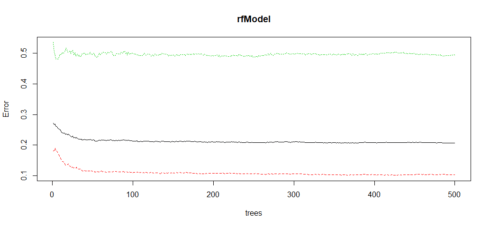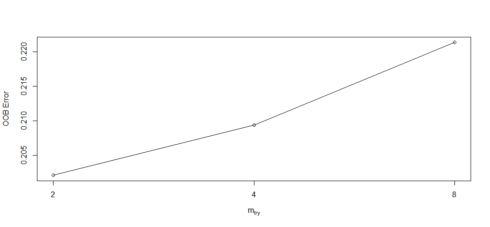Want to share your content on R-bloggers? click here if you have a blog, or here if you don't.

Customer churn occurs when customers or subscribers stop doing business with a company or service, also known as customer attrition. It is also referred as loss of clients or customers. One industry in which churn rates are particularly useful is the telecommunications industry, because most customers have multiple options from which to choose within a geographic location.

Similar concept with predicting employee turnover, we are going to predict customer churn using telecom dataset. We will introduce Logistic Regression, Decision Tree, and Random Forest. But this time, we will do all of the above in R. Let’s get started!

## Data Preprocessing

The data was downloaded from IBM Sample Data Sets. Each row represents a customer, each column contains that customer’s attributes:

```library(plyr)
library(corrplot)
library(ggplot2)
library(gridExtra)
library(ggthemes)
library(caret)
library(MASS)
library(randomForest)
library(party)

str(churn)
'data.frame':	7043 obs. of  21 variables:
\$ customerID      : Factor w/ 7043 levels "0002-ORFBO","0003-MKNFE",..: 5376 3963 2565 5536 6512 6552 1003 4771 5605 4535 ...
\$ gender          : Factor w/ 2 levels "Female","Male": 1 2 2 2 1 1 2 1 1 2 ...
\$ SeniorCitizen   : int  0 0 0 0 0 0 0 0 0 0 ...
\$ Partner         : Factor w/ 2 levels "No","Yes": 2 1 1 1 1 1 1 1 2 1 ...
\$ Dependents      : Factor w/ 2 levels "No","Yes": 1 1 1 1 1 1 2 1 1 2 ...
\$ tenure          : int  1 34 2 45 2 8 22 10 28 62 ...
\$ PhoneService    : Factor w/ 2 levels "No","Yes": 1 2 2 1 2 2 2 1 2 2 ...
\$ MultipleLines   : Factor w/ 3 levels "No","No phone service",..: 2 1 1 2 1 3 3 2 3 1 ...
\$ InternetService : Factor w/ 3 levels "DSL","Fiber optic",..: 1 1 1 1 2 2 2 1 2 1 ...
\$ OnlineSecurity  : Factor w/ 3 levels "No","No internet service",..: 1 3 3 3 1 1 1 3 1 3 ...
\$ OnlineBackup    : Factor w/ 3 levels "No","No internet service",..: 3 1 3 1 1 1 3 1 1 3 ...
\$ DeviceProtection: Factor w/ 3 levels "No","No internet service",..: 1 3 1 3 1 3 1 1 3 1 ...
\$ TechSupport     : Factor w/ 3 levels "No","No internet service",..: 1 1 1 3 1 1 1 1 3 1 ...
\$ StreamingTV     : Factor w/ 3 levels "No","No internet service",..: 1 1 1 1 1 3 3 1 3 1 ...
\$ StreamingMovies : Factor w/ 3 levels "No","No internet service",..: 1 1 1 1 1 3 1 1 3 1 ...
\$ Contract        : Factor w/ 3 levels "Month-to-month",..: 1 2 1 2 1 1 1 1 1 2 ...
\$ PaperlessBilling: Factor w/ 2 levels "No","Yes": 2 1 2 1 2 2 2 1 2 1 ...
\$ PaymentMethod   : Factor w/ 4 levels "Bank transfer (automatic)",..: 3 4 4 1 3 3 2 4 3 1 ...
\$ MonthlyCharges  : num  29.9 57 53.9 42.3 70.7 ...
\$ TotalCharges    : num  29.9 1889.5 108.2 1840.8 151.7 ...
\$ Churn           : Factor w/ 2 levels "No","Yes": 1 1 2 1 2 2 1 1 2 1 ...

```

The raw data contains 7043 rows (customers) and 21 columns (features). The “Churn” column is our target.

We use sapply to check the number if missing values in each columns. We found that there are 11 missing values in “TotalCharges” columns. So, let’s remove all rows with missing values.

```sapply(churn, function(x) sum(is.na(x)))
customerID           gender    SeniorCitizen          Partner
0                0                0                0
Dependents           tenure     PhoneService    MultipleLines
0                0                0                0
InternetService   OnlineSecurity     OnlineBackup DeviceProtection
0                0                0                0
TechSupport      StreamingTV  StreamingMovies         Contract
0                0                0                0
PaperlessBilling    PaymentMethod   MonthlyCharges     TotalCharges
0                0                0               11
Churn
0

churn <- churn[complete.cases(churn), ]
```

### Look at the variables, we can see that we have some wranglings to do.

1. We will change “No internet service” to “No” for six columns, they are: “OnlineSecurity”, “OnlineBackup”, “DeviceProtection”, “TechSupport”, “streamingTV”, “streamingMovies”.

```cols_recode1 <- c(10:15)
for(i in 1:ncol(churn[,cols_recode1])) {
churn[,cols_recode1][,i] <- as.factor(mapvalues
(churn[,cols_recode1][,i], from =c("No internet service"),to=c("No")))
}
```

2. We will change “No phone service” to “No” for column “MultipleLines”

```churn\$MultipleLines <- as.factor(mapvalues(churn\$MultipleLines,
from=c("No phone service"),
to=c("No")))
```

3. Since the minimum tenure is 1 month and maximum tenure is 72 months, we can group them into five tenure groups: “0–12 Month”, “12–24 Month”, “24–48 Months”, “48–60 Month”, “> 60 Month”

```min(churn\$tenure); max(churn\$tenure)
 1
 72

group_tenure = 0 & tenure  12 & tenure  24 & tenure  48 & tenure  60){
return('> 60 Month')
}
}
churn\$tenure_group <- sapply(churn\$tenure,group_tenure)
churn\$tenure_group <- as.factor(churn\$tenure_group)
```

4. Change the values in column “SeniorCitizen” from 0 or 1 to “No” or “Yes”.

```churn\$SeniorCitizen <- as.factor(mapvalues(churn\$SeniorCitizen,
from=c("0","1"),
to=c("No", "Yes")))
```

5. Remove the columns we do not need for the analysis.

```churn\$customerID <- NULL
churn\$tenure <- NULL
```

## Exploratory data analysis and feature selection

Correlation between numeric variables

```numeric.var <- sapply(churn, is.numeric)
corr.matrix <- cor(churn[,numeric.var])
corrplot(corr.matrix, main="\n\nCorrelation Plot for Numerical Variables", method="number")
```

Gives this plot:The Monthly Charges and Total Charges are correlated. So one of them will be removed from the model. We remove Total Charges.

```churn\$TotalCharges <- NULL
```

Bar plots of categorical variables

```p1 <- ggplot(churn, aes(x=gender)) + ggtitle("Gender") + xlab("Gender") +
geom_bar(aes(y = 100*(..count..)/sum(..count..)), width = 0.5) + ylab("Percentage") + coord_flip() + theme_minimal()
p2 <- ggplot(churn, aes(x=SeniorCitizen)) + ggtitle("Senior Citizen") + xlab("Senior Citizen") +
geom_bar(aes(y = 100*(..count..)/sum(..count..)), width = 0.5) + ylab("Percentage") + coord_flip() + theme_minimal()
p3 <- ggplot(churn, aes(x=Partner)) + ggtitle("Partner") + xlab("Partner") +
geom_bar(aes(y = 100*(..count..)/sum(..count..)), width = 0.5) + ylab("Percentage") + coord_flip() + theme_minimal()
p4 <- ggplot(churn, aes(x=Dependents)) + ggtitle("Dependents") + xlab("Dependents") +
geom_bar(aes(y = 100*(..count..)/sum(..count..)), width = 0.5) + ylab("Percentage") + coord_flip() + theme_minimal()
grid.arrange(p1, p2, p3, p4, ncol=2)
```
```p5 <- ggplot(churn, aes(x=PhoneService)) + ggtitle("Phone Service") + xlab("Phone Service") +
geom_bar(aes(y = 100*(..count..)/sum(..count..)), width = 0.5) + ylab("Percentage") + coord_flip() + theme_minimal()
p6 <- ggplot(churn, aes(x=MultipleLines)) + ggtitle("Multiple Lines") + xlab("Multiple Lines") +
geom_bar(aes(y = 100*(..count..)/sum(..count..)), width = 0.5) + ylab("Percentage") + coord_flip() + theme_minimal()
p7 <- ggplot(churn, aes(x=InternetService)) + ggtitle("Internet Service") + xlab("Internet Service") +
geom_bar(aes(y = 100*(..count..)/sum(..count..)), width = 0.5) + ylab("Percentage") + coord_flip() + theme_minimal()
p8 <- ggplot(churn, aes(x=OnlineSecurity)) + ggtitle("Online Security") + xlab("Online Security") +
geom_bar(aes(y = 100*(..count..)/sum(..count..)), width = 0.5) + ylab("Percentage") + coord_flip() + theme_minimal()
grid.arrange(p5, p6, p7, p8, ncol=2)
```
```p9 <- ggplot(churn, aes(x=OnlineBackup)) + ggtitle("Online Backup") + xlab("Online Backup") +
geom_bar(aes(y = 100*(..count..)/sum(..count..)), width = 0.5) + ylab("Percentage") + coord_flip() + theme_minimal()
p10 <- ggplot(churn, aes(x=DeviceProtection)) + ggtitle("Device Protection") + xlab("Device Protection") +
geom_bar(aes(y = 100*(..count..)/sum(..count..)), width = 0.5) + ylab("Percentage") + coord_flip() + theme_minimal()
p11 <- ggplot(churn, aes(x=TechSupport)) + ggtitle("Tech Support") + xlab("Tech Support") +
geom_bar(aes(y = 100*(..count..)/sum(..count..)), width = 0.5) + ylab("Percentage") + coord_flip() + theme_minimal()
p12 <- ggplot(churn, aes(x=StreamingTV)) + ggtitle("Streaming TV") + xlab("Streaming TV") +
geom_bar(aes(y = 100*(..count..)/sum(..count..)), width = 0.5) + ylab("Percentage") + coord_flip() + theme_minimal()
grid.arrange(p9, p10, p11, p12, ncol=2)
```
```p13 <- ggplot(churn, aes(x=StreamingMovies)) + ggtitle("Streaming Movies") + xlab("Streaming Movies") +
geom_bar(aes(y = 100*(..count..)/sum(..count..)), width = 0.5) + ylab("Percentage") + coord_flip() + theme_minimal()
p14 <- ggplot(churn, aes(x=Contract)) + ggtitle("Contract") + xlab("Contract") +
geom_bar(aes(y = 100*(..count..)/sum(..count..)), width = 0.5) + ylab("Percentage") + coord_flip() + theme_minimal()
p15 <- ggplot(churn, aes(x=PaperlessBilling)) + ggtitle("Paperless Billing") + xlab("Paperless Billing") +
geom_bar(aes(y = 100*(..count..)/sum(..count..)), width = 0.5) + ylab("Percentage") + coord_flip() + theme_minimal()
p16 <- ggplot(churn, aes(x=PaymentMethod)) + ggtitle("Payment Method") + xlab("Payment Method") +
geom_bar(aes(y = 100*(..count..)/sum(..count..)), width = 0.5) + ylab("Percentage") + coord_flip() + theme_minimal()
p17 <- ggplot(churn, aes(x=tenure_group)) + ggtitle("Tenure Group") + xlab("Tenure Group") +
geom_bar(aes(y = 100*(..count..)/sum(..count..)), width = 0.5) + ylab("Percentage") + coord_flip() + theme_minimal()
grid.arrange(p13, p14, p15, p16, p17, ncol=2)
```

All of the categorical variables seem to have a reasonably broad distribution, therefore, all of them will be kept for the further analysis.

## Logistic Regression

First, we split the data into training and testing sets

```intrain<- createDataPartition(churn\$Churn,p=0.7,list=FALSE)
set.seed(2017)
training<- churn[intrain,]
testing<- churn[-intrain,]
```

Confirm the splitting is correct

```dim(training); dim(testing)
 4924   19
 2108   19

```

Fitting the Logistic Regression Model

```LogModel <- glm(Churn ~ .,family=binomial(link="logit"),data=training)
print(summary(LogModel))
Call:
glm(formula = Churn ~ ., family = binomial(link = "logit"), data = training)

Deviance Residuals:
Min       1Q   Median       3Q      Max
-2.0370  -0.6793  -0.2861   0.6590   3.1608

Coefficients:
Estimate Std. Error z value Pr(>|z|)
(Intercept)                          -2.030149   1.008223  -2.014 0.044053 *
genderMale                           -0.039006   0.077686  -0.502 0.615603
SeniorCitizenYes                      0.194408   0.101151   1.922 0.054611 .
PartnerYes                           -0.062031   0.092911  -0.668 0.504363
DependentsYes                        -0.017974   0.107659  -0.167 0.867405
PhoneServiceYes                      -0.387097   0.788745  -0.491 0.623585
MultipleLinesYes                      0.345052   0.214799   1.606 0.108187
InternetServiceFiber optic            1.022836   0.968062   1.057 0.290703
InternetServiceNo                    -0.829521   0.978909  -0.847 0.396776
OnlineSecurityYes                    -0.393647   0.215993  -1.823 0.068379 .
OnlineBackupYes                      -0.113220   0.213825  -0.529 0.596460
DeviceProtectionYes                  -0.025797   0.213317  -0.121 0.903744
TechSupportYes                       -0.306423   0.220920  -1.387 0.165433
StreamingTVYes                        0.399209   0.395912   1.008 0.313297
StreamingMoviesYes                    0.338852   0.395890   0.856 0.392040
ContractOne year                     -0.805584   0.127125  -6.337 2.34e-10 ***
ContractTwo year                     -1.662793   0.216346  -7.686 1.52e-14 ***
PaperlessBillingYes                   0.338724   0.089407   3.789 0.000152 ***
PaymentMethodCredit card (automatic) -0.018574   0.135318  -0.137 0.890826
PaymentMethodElectronic check         0.373214   0.113029   3.302 0.000960 ***
PaymentMethodMailed check             0.001400   0.136446   0.010 0.991815
MonthlyCharges                       -0.005001   0.038558  -0.130 0.896797
tenure_group0-12 Month                1.858899   0.205956   9.026  < 2e-16 ***
tenure_group12-24 Month               0.968497   0.201452   4.808 1.53e-06 ***
tenure_group24-48 Month               0.574822   0.185500   3.099 0.001943 **
tenure_group48-60 Month               0.311790   0.200096   1.558 0.119185
---
Signif. codes:  0 ‘***’ 0.001 ‘**’ 0.01 ‘*’ 0.05 ‘.’ 0.1 ‘ ’ 1

(Dispersion parameter for binomial family taken to be 1)

Null deviance: 5702.8  on 4923  degrees of freedom
Residual deviance: 4094.4  on 4898  degrees of freedom
AIC: 4146.4

Number of Fisher Scoring iterations: 6

```

Feature Analysis
The top three most-relevant features include Contract, tenure_group and PaperlessBilling.

```anova(LogModel, test="Chisq")
Analysis of Deviance Table
Response: Churn
Terms added sequentially (first to last)
Df Deviance Resid. Df Resid. Dev  Pr(>Chi)
NULL                              4923     5702.8
gender            1     0.39      4922     5702.4 0.5318602
SeniorCitizen     1    95.08      4921     5607.3 < 2.2e-16 ***
Partner           1   107.29      4920     5500.0 < 2.2e-16 ***
Dependents        1    27.26      4919     5472.7 1.775e-07 ***
PhoneService      1     1.27      4918     5471.5 0.2597501
MultipleLines     1     9.63      4917     5461.8 0.0019177 **
InternetService   2   452.01      4915     5009.8 < 2.2e-16 ***
OnlineSecurity    1   183.83      4914     4826.0 < 2.2e-16 ***
OnlineBackup      1    69.94      4913     4756.1 < 2.2e-16 ***
DeviceProtection  1    47.58      4912     4708.5 5.287e-12 ***
TechSupport       1    82.78      4911     4625.7 < 2.2e-16 ***
StreamingTV       1     4.90      4910     4620.8 0.0269174 *
StreamingMovies   1     0.36      4909     4620.4 0.5461056
Contract          2   309.25      4907     4311.2 < 2.2e-16 ***
PaperlessBilling  1    14.21      4906     4297.0 0.0001638 ***
PaymentMethod     3    38.85      4903     4258.1 1.867e-08 ***
MonthlyCharges    1     0.10      4902     4258.0 0.7491553
tenure_group      4   163.67      4898     4094.4 < 2.2e-16 ***
---
Signif. codes:  0 ‘***’ 0.001 ‘**’ 0.01 ‘*’ 0.05 ‘.’ 0.1 ‘ ’ 1

```

Analyzing the deviance table we can see the drop in deviance when adding each variable one at a time. Adding InternetService, Contract and tenure_group significantly reduces the residual deviance. The other variables such as PaymentMethod and Dependents seem to improve the model less even though they all have low p-values.

Assessing the predictive ability of the Logistic Regression model

```testing\$Churn <- as.character(testing\$Churn)
testing\$Churn[testing\$Churn=="No"] <- "0"
testing\$Churn[testing\$Churn=="Yes"] <- "1"
fitted.results <- predict(LogModel,newdata=testing,type='response')
fitted.results  0.5,1,0)
misClasificError <- mean(fitted.results != testing\$Churn)
print(paste('Logistic Regression Accuracy',1-misClasificError))
 "Logistic Regression Accuracy 0.796489563567362"

```

Logistic Regression Confusion Matrix

```print("Confusion Matrix for Logistic Regression"); table(testing\$Churn, fitted.results > 0.5)
 "Confusion Matrix for Logistic Regression"

FALSE TRUE
0  1392  156
1   273  287

```

Odds Ratio
One of the interesting performance measurements in logistic regression is Odds Ratio.Basically, Odds ratio is what the odds of an event is happening.

```exp(cbind(OR=coef(LogModel), confint(LogModel)))
Waiting for profiling to be done...
OR      2.5 %     97.5 %
(Intercept)                          0.1313160 0.01815817  0.9461855
genderMale                           0.9617454 0.82587632  1.1199399
SeniorCitizenYes                     1.2145919 0.99591418  1.4807053
PartnerYes                           0.9398537 0.78338071  1.1276774
DependentsYes                        0.9821863 0.79488224  1.2124072
PhoneServiceYes                      0.6790251 0.14466019  3.1878587
MultipleLinesYes                     1.4120635 0.92707245  2.1522692
InternetServiceFiber optic           2.7810695 0.41762316 18.5910286
InternetServiceNo                    0.4362582 0.06397364  2.9715699
OnlineSecurityYes                    0.6745919 0.44144273  1.0296515
OnlineBackupYes                      0.8929545 0.58709919  1.3577947
DeviceProtectionYes                  0.9745328 0.64144877  1.4805460
TechSupportYes                       0.7360754 0.47707096  1.1344691
StreamingTVYes                       1.4906458 0.68637788  3.2416264
StreamingMoviesYes                   1.4033353 0.64624171  3.0518161
ContractOne year                     0.4468271 0.34725066  0.5717469
ContractTwo year                     0.1896086 0.12230199  0.2861341
PaperlessBillingYes                  1.4031556 1.17798691  1.6725920
PaymentMethodCredit card (automatic) 0.9815977 0.75273387  1.2797506
PaymentMethodElectronic check        1.4523952 1.16480721  1.8145076
PaymentMethodMailed check            1.0014007 0.76673087  1.3092444
MonthlyCharges                       0.9950112 0.92252949  1.0731016
tenure_group0-12 Month               6.4166692 4.30371945  9.6544837
tenure_group12-24 Month              2.6339823 1.78095906  3.9256133
tenure_group24-48 Month              1.7768147 1.23988035  2.5676783
tenure_group48-60 Month              1.3658675 0.92383315  2.0261505

```

## Decision Tree

Decision Tree visualization
For illustration purpose, we are going to use only three variables for plotting Decision Trees, they are “Contract”, “tenure_group” and “PaperlessBilling”.

```tree <- ctree(Churn~Contract+tenure_group+PaperlessBilling, training)
plot(tree, type='simple')
```

Gives this plot:1. Out of three variables we use, Contract is the most important variable to predict customer churn or not churn.
2. If a customer in a one-year or two-year contract, no matter he (she) has PapelessBilling or not, he (she) is less likely to churn.
3. On the other hand, if a customer is in a month-to-month contract, and in the tenure group of 0–12 month, and using PaperlessBilling, then this customer is more likely to churn.

Decision Tree Confusion Matrix
We are using all the variables to product confusion matrix table and make predictions.

```pred_tree <- predict(tree, testing)
print("Confusion Matrix for Decision Tree"); table(Predicted = pred_tree, Actual = testing\$Churn)
 "Confusion Matrix for Decision Tree"
Actual
Predicted   No  Yes
No  1395  346
Yes  153  214

```

Decision Tree Accuracy

```p1 <- predict(tree, training)
tab1 <- table(Predicted = p1, Actual = training\$Churn)
tab2 <- table(Predicted = pred_tree, Actual = testing\$Churn)
print(paste('Decision Tree Accuracy',sum(diag(tab2))/sum(tab2)))
 "Decision Tree Accuracy 0.763282732447818"

```

The accuracy for Decision Tree has hardly improved. Let’s see if we can do better using Random Forest.

## Random Forest

Random Forest Initial Model

```rfModel <- randomForest(Churn ~., data = training)
print(rfModel)
Call:
randomForest(formula = Churn ~ ., data = training)
Type of random forest: classification
Number of trees: 500
No. of variables tried at each split: 4

OOB estimate of  error rate: 20.65%
Confusion matrix:
No Yes class.error
No  3245 370   0.1023513
Yes  647 662   0.4942704

```

The error rate is relatively low when predicting “No”, and the error rate is much higher when predicting “Yes”.

Random Forest Prediction and Confusion Matrix

```pred_rf <- predict(rfModel, testing)
caret::confusionMatrix(pred_rf, testing\$Churn)
Confusion Matrix and Statistics

Reference
Prediction   No  Yes
No  1381  281
Yes  167  279

Accuracy : 0.7875
95% CI : (0.7694, 0.8048)
No Information Rate : 0.7343
P-Value [Acc > NIR] : 9.284e-09

Kappa : 0.4175
Mcnemar's Test P-Value : 9.359e-08

Sensitivity : 0.8921
Specificity : 0.4982
Pos Pred Value : 0.8309
Neg Pred Value : 0.6256
Prevalence : 0.7343
Detection Rate : 0.6551
Detection Prevalence : 0.7884
Balanced Accuracy : 0.6952

'Positive' Class : No

```

Random Forest Error Rate

```plot(rfModel)
```

Gives this plot:We use this plot to help us determine the number of trees. As the number of trees increases, the OOB error rate decreases, and then becomes almost constant. We are not able to decrease the OOB error rate after about 100 to 200 trees.

Tune Random Forest Model

```t <- tuneRF(training[, -18], training[, 18], stepFactor = 0.5, plot = TRUE, ntreeTry = 200, trace = TRUE, improve = 0.05)
```

Gives this plot:We use this plot to give us some ideas on the number of mtry to choose. OOB error rate is at the lowest when mtry is 2. Therefore, we choose mtry=2.

Fit the Random Forest Model After Tuning

```rfModel_new <- randomForest(Churn ~., data = training, ntree = 200, mtry = 2, importance = TRUE, proximity = TRUE)
print(rfModel_new)
Call:
randomForest(formula = Churn ~ ., data = training, ntree = 200,      mtry = 2, importance = TRUE, proximity = TRUE)
Type of random forest: classification
Number of trees: 200
No. of variables tried at each split: 2

OOB estimate of  error rate: 19.7%
Confusion matrix:
No Yes class.error
No  3301 314   0.0868603
Yes  656 653   0.5011459

```

OOB error rate decreased to 19.7% from 20.65% earlier.

Random Forest Predictions and Confusion Matrix After Tuning

```pred_rf_new <- predict(rfModel_new, testing)
caret::confusionMatrix(pred_rf_new, testing\$Churn)
Confusion Matrix and Statistics

Reference
Prediction   No  Yes
No  1404  305
Yes  144  255

Accuracy : 0.787
95% CI : (0.7689, 0.8043)
No Information Rate : 0.7343
P-Value [Acc > NIR] : 1.252e-08

Kappa : 0.3989
Mcnemar's Test P-Value : 4.324e-14

Sensitivity : 0.9070
Specificity : 0.4554
Pos Pred Value : 0.8215
Neg Pred Value : 0.6391
Prevalence : 0.7343
Detection Rate : 0.6660
Detection Prevalence : 0.8107
Balanced Accuracy : 0.6812

'Positive' Class : No

```

The accuracy did not increase but the sensitivity improved, compare with the initial Random Forest model.

Random Forest Feature Importance

```varImpPlot(rfModel_new, sort=T, n.var = 10, main = 'Top 10 Feature Importance')
```

## Summary

From the above example, we can see that Logistic Regression and Random Forest performed better than Decision Tree for customer churn analysis for this particular dataset.

Throughout the analysis, I have learned several important things:
1. Features such as tenure_group, Contract, PaperlessBilling, MonthlyCharges and InternetService appear to play a role in customer churn.
2. There does not seem to be a relationship between gender and churn.
3. Customers in a month-to-month contract, with PaperlessBilling and are within 12 months tenure, are more likely to churn; On the other hand, customers with one or two year contract, with longer than 12 months tenure, that are not using PaperlessBilling, are less likely to churn.

Source code that created this post can be found here. I would be pleased to receive feedback or questions on any of the above.

Related Post